# Capitalized Income Approach

The Capitalized Income Approach, or direct capitalization Income Approach, is a valuation method used for real estate. There are two approaches that fall under the Income approach, the direct capitalization approach and the discounted cash flow method.

On this page, we focus on the direct capitalization method. In particular, we discuss the calculation of the capitalization rate (CP) and net operating income (NOI). Both metrics are needed to apply the Income approach. A spreadsheet at the bottom of the page provides a capitalized income approach calculator.

## Capitalized Income approach definition

Value under the Income approach is based on net operating income (NOI). NOI has to be calculate first before we can apply the income approach. To calculate NOI, we first calculate rental income when the building is fully occupied. Next, we add other income, subtract vacancy and collection losses, and operating expenses. Operating expenses include insurance, property taxes, utilities, maintenance, and repairs. It is important to keep in mind we need the forecasted NOI, that is, NOI for next year.

The second ingredient is the capitalization rate. The capitalization rate is typically calculated using data on comparable sales. In particular, we collect the sales prices of other properties as well as the NOI of these properties. Then, we can calculate the cap rate as follows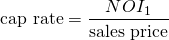If we have the discount rate and the expected growth rate of NOI, we can also calculate the cap rate as follows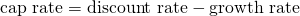Once we have the cap rate and NOI, we can calculate the value as follows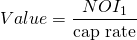## Income approach examples

Let’s have a look at and example. The following table considers an example of a property building. In particular, let’s have a look at a shopping centre. Note that the table reports more data than we actually need.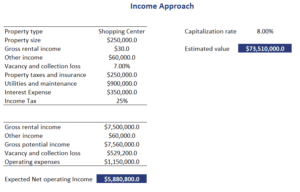## All Risks yield

Sometimes, the tenant pays all the expenses. In that case, we can use the rent directly because it reflects NOI. In that case, the cap rate is called the all risks yield. Calculating the value using an all risks yield is similar to the income approach. In particular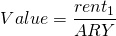## Summary

We discussed the income approach. A commonly used method to value commercial real estate. While the approach is easy to use, there is one caveat. Sometimes, investors have to adjust NOI such that it is not influenced by temporary effects. In that case, we first need to calculate Stabilized NOI.